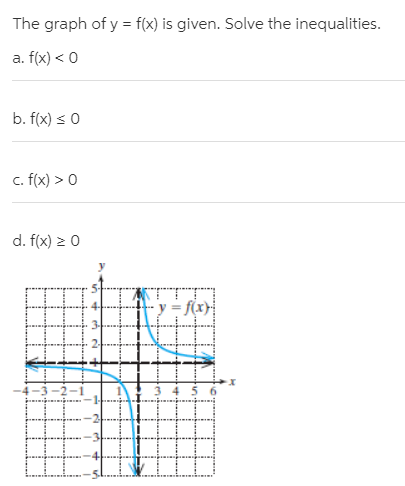# The graph of y = f(x) is given. Solve the inequalities. a. f(x) < 0 b. f(x) s 0 c. f(x) > 0 d. f(x) > 0 y = f(x)

Questionhelp_outlineImage TranscriptioncloseThe graph of y = f(x) is given. Solve the inequalities. a. f(x) < 0 b. f(x) s 0 c. f(x) > 0 d. f(x) > 0 y = f(x) fullscreen